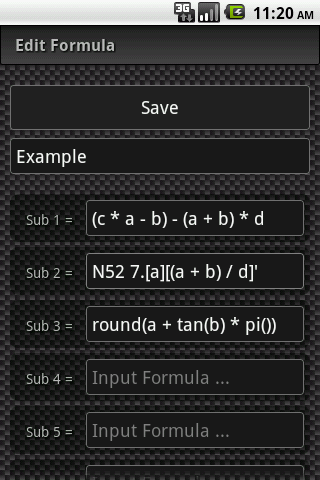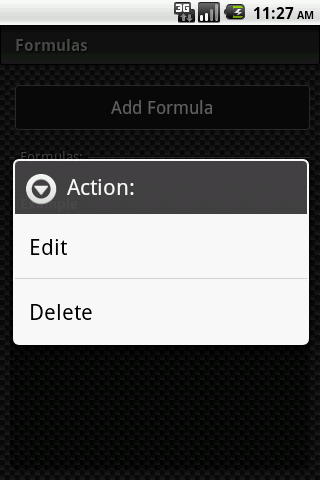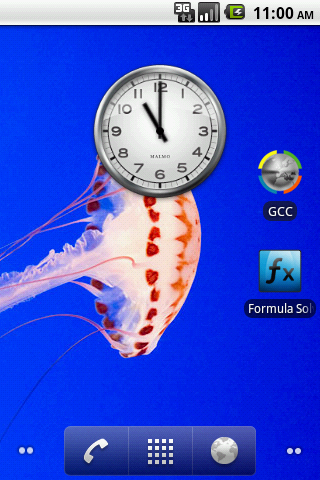﻿ GCC - GeoCache Calculator
The GeoCache Calculator is a comprehensive tool for every active cacher! It contains different functions to calculate values and checksums, as well as tables and overviews for supporting your outdoor geocaching search. A tool which no cacher should miss!

# 1. Formula Solver

The in GCC contained formula solver helps you to solve complex calculations, easily on your outdoor cachung tour. You got different functions with this tool, wich you may solve every cache and calculation out there!

## 2. Usage

The formula solver is seperated in two aeras. The values and the formulas. Of course you can insert numbers directly into your formulas. But while solving geocaches, you have often to calculate with letter. GCC could even do this for you. For this you have to add a new formula to calculate. As an example:
"(c * a - b) - (a + b) * d"Inside the values area you can set the value for each letter in your formulas. If no value is set for an letter, the default value of it is "0" (zero). Example values:

A = 6
B = 4
C = 8
D = 2

The formula solver now replace all values in your formula and gives you the result. As in your example, the solver calculates: "(8 * 6 - 4) - (6 + 4) * 2" with the result "24".

## 3. Edit and delete

If you have to edit or even delete one of your formulas, you could do this over the context menu inside the formula list. Just hold your finger on the formula, you wish to edit or delete. An dialog is now shown on the screen, wich allows you to edit or delete the formula directly.In the values area you could easily edit or change a single value as you want. But you even can reset all values at once. Just click on your menu button on the device an select "reset values".

## 4. Replacement of Values

It is also possible to put your own text or the rest of the coordinates inside the formula fields. For this you have to put all your values letters and calculations inside of squared brackets, to replace them. As example: "N 52º 7.[a][(a + b) / d]'"

Now the contents of the squared brackets would be replaced with the result and shown in your text. So the output of the formula above would be: "N 52º 7.65'"

## 5. Widget

When you use the formula solver at your caching tour, would it in certain circumstances a bit annoying, that you have to open GCC first, to get into the formula solver. Because of this, we have invented the Widgets for GCC. With this little helpers, you can put a link to the formula solver directly on the home screen of your android device.

For this you have to search for an empty place on you screen an hold your finger there. In the opening dialog you have to select "widgets" and select "GCC Formula Solver". After this you have an direct link to easy and fast access the formula solver inside of GCC.## 6. Included Functions

Of course GCC have different math functions, to support you at many calculations you have to solve. This function could be insert directly into your formulas. Example: "round(a + tan(b) * pi())" Here you get a list of all includet function you can use:

No or more Parameters
- min, max

One or more Parameters
- avg
- sum

No Parameters
- e
- pi
- rand

One Parameter
- abs, acos, acosh, asin, asinh, atan, atanh
- ceil, cos, cosh
- exp
- fact, floor
- lg, ln
- round
- sign, sin, sinh, sqrt
- tan, tanh

Two Parameters
- combin
- mod
- pow

One or Two Parameters
- log